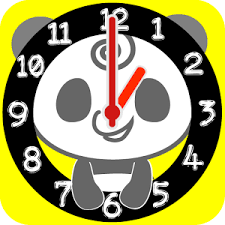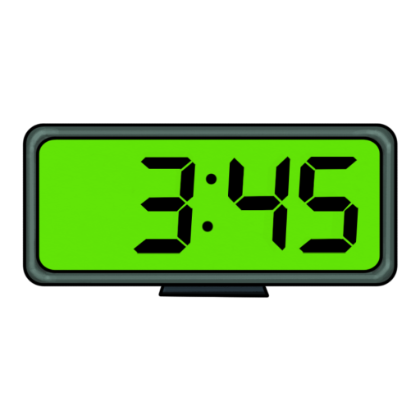# 2nd Grade Math Vocabulary Flashcards

2nd Grade Math Vocabulary Flashcards
1/16 (missed) 0 0
Create Your Account To Continue Studying

As a member, you'll also get unlimited access to over 79,000 lessons in math, English, science, history, and more. Plus, get practice tests, quizzes, and personalized coaching to help you succeed.

Try it risk-free for 30 days. Cancel anytime
Odd
A number that cannot be equally divided and has a leftover part is called an odd number. Examples of an odd number would be 1, 3, 5, 15, 27, and 79.
Got it
Numeral
A number that can contain multiple digits is called a numeral. An example of a numeral is 362, which consists of three digits.
Got it
Digit
A single numeral between zero and nine is called a digit.
Got it
Integer
A whole number that is not a fraction or a decimal is called an integer.
Got it
Hundreds
The number position to the immediate left of the tens place is the hundreds place. In the number 7942, 9 is in the hundreds place.
Got it
Tens
The number position to the immediate left of the ones place is the tens place. In the number 7942, 4 is in the tens place.
Got it
Ones
The number position to the immediate left of the decimal point is the ones place. In the number 7942, 2 is in the ones place.
Got it
Place Value
The position in a series of numbers is the place value of the number.
Got it

### Ready to move on?

or choose a specific lesson: See all lessons in this chapter
16 cards in set

## Flashcard Content Overview

This flashcard set covers the vocabulary words that you will use in your 2nd grade Math class. They will help you understand the math terms that you will encounter as you learn numbers. It would be best for you to learn these definitions. They will prove to be very useful in your future lessons.

Front
Back
Place Value
The position in a series of numbers is the place value of the number.
Ones
The number position to the immediate left of the decimal point is the ones place. In the number 7942, 2 is in the ones place.
Tens
The number position to the immediate left of the ones place is the tens place. In the number 7942, 4 is in the tens place.
Hundreds
The number position to the immediate left of the tens place is the hundreds place. In the number 7942, 9 is in the hundreds place.
Integer
A whole number that is not a fraction or a decimal is called an integer.
Digit
A single numeral between zero and nine is called a digit.
Numeral
A number that can contain multiple digits is called a numeral. An example of a numeral is 362, which consists of three digits.
Odd
A number that cannot be equally divided and has a leftover part is called an odd number. Examples of an odd number would be 1, 3, 5, 15, 27, and 79.
Even
A number that can be equally divided with no leftover parts is an even number. Examples of an even number would be 2, 4, 8, 14, 36, and 72.
Equivalent
Items that are equal are also equivalent. Fractions can be compared as equivalent.
Equal Parts
An item that is divided correctly is said to be in equal parts. Fractions can have equal parts.
Whole
Something that is complete or not missing any pieces. A number that has no fraction or decimal is called a whole number. Examples would be 7, 25, and 168. The number 53.9 is not a whole number.
A number that is added to another number is called an addend.
Doubles
If something has two of the same numbers, it is called doubles. You can add doubles, such as 2 + 2. You can also subtract doubles, such as 7 - 7. Any math operation can be performed with doubles.
Analog Clock
A clock with hands showing the hour and minute, and possibly the seconds, is called an analog clock.Digital Clock
A clock that only tells time with numbers is called a digital clock.To unlock this flashcard set you must be a Study.com Member.